IF YOU CAN SEE THIS go to /sfa/Nuprl/Shared/Xindentation_hack_doc.html
DiscreteMath
Nuprl Section: DiscreteMath - Discrete Math Lessons
Counting is finding a function of a certain kind.
When we count a class of objects, we generate an enumeration of them, which we may represent by a One-to-One Correspondence from a standard class having that many objects to the class being counted. Our standard class of n objects, for n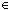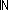, will ben, which is the class {k: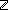| 0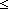k < n } of natural numbers less than n. A more familiar choice of standard finite classes might have been {k:| 1kn }, but there is also another tradition in math for using {k:| 0k < n }.

So, a class A has n members just when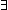f:(n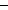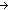A). Bij(nAf)

which may also be expressed as

(n ~ A)

since Thm*  (f:(AB). Bij(ABf))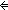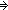(A ~ B), or as

(A ~n) since Thm*  (A ~ B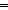(B ~ A).

Now, since counting means coming up with an enumeration, we may ask whether counting in different ways, i.e., coming up with different orders, will always result in the same number, as we assume. Of course, we know this is so, but there are different degrees of knowing. It is not necessary to simply accept this as an axiom; there is enough structure to the problem to make a non-trivial proof.

Thm*  (A ~m(A ~km = k

This theorem is closely related to what is sometimes called the "pigeon-hole principle", which states the mathematical content of the fact that if you put some number objects into fewer pigeon-holes, then there must be at least two objects going into the same pigeon-hole. Number the pigeon-holes with the members ofk, and the objects with the members ofm; then a way of putting the objects into the holes is a function inmk:

Thm*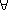m,k:f:(mk). k<m(x,y:mx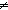y & f(x) = f(y))

If you examine the proofs of these theorems, you will notice that they both cite the key lemma

Thm*  (f:(mk). Inj(mkf))mk.

Counting Indirectly is a strategy more common than explicitly describing a counting function for a problem.